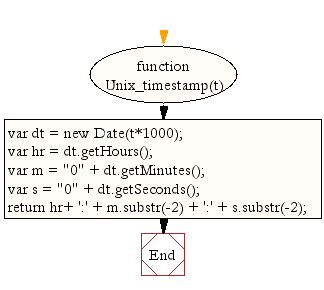# JavaScript: Convert a Unix timestamp to time

## JavaScript Datetime: Exercise-17 with Solution

Write a JavaScript function to convert a Unix timestamp to time.

Test Data:
console.log(Unix_timestamp(1412743274));
10:11:14

Sample Solution:-

HTML Code:

``````<!DOCTYPE html>
<html>
<meta charset="utf-8">
<title>JavaScript function to convert a Unix timestamp to time.</title>
<body>

</body>
</html>
```
```

JavaScript Code:

``````function Unix_timestamp(t)
{
var dt = new Date(t*1000);
var hr = dt.getHours();
var m = "0" + dt.getMinutes();
var s = "0" + dt.getSeconds();
return hr+ ':' + m.substr(-2) + ':' + s.substr(-2);
}

console.log(Unix_timestamp(1412743274));
```
```

Sample Output:

```10:11:14
```

Flowchart:Live Demo:

See the Pen JavaScript - Convert a Unix timestamp to time-date-ex- 17 by w3resource (@w3resource) on CodePen.

Improve this sample solution and post your code through Disqus

What is the difficulty level of this exercise?

﻿

## JavaScript: Tips of the Day

|| operator

```const one = false || {} || null;
const two = null || false || '';
const three = [] || 0 || true;

console.log(one, two, three);
```

With the || operator, we can return the first truthy operand. If all values are falsy, the last operand gets returned.
(false || {} || null): the empty object {} is a truthy value. This is the first (and only) truthy value, which gets returned. one is equal to {}.
(null || false || ""): all operands are falsy values. This means that the last operand, "" gets returned. two is equal to "".
([] || 0 || ""): the empty array[] is a truthy value. This is the first truthy value, which gets returned. three is equal to [].

Ref: https://bit.ly/3jFRBje